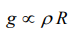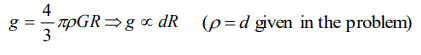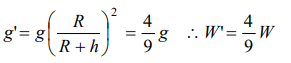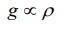## Gravitation Questions and Answers Part-9

1. If density of earth increased 4 times and its radius become half of what it is, our weight will
a) Be four times its present value
b) Be doubled
c) Remain same
d) Be halved

Explanation:2. A man can jump to a height of 1.5 m on a planet A. What is the height he may be able to jump on another planet whose density and radius are,respectively, one-quarter and one-third that of planet A
a) 1.5 m
b) 15 m
c) 18 m
d) 28 m

Explanation:3. Weight of a body is maximum at
a) Moon
b) Poles of earth
c) Equator of earth
d) Centre of earth

Explanation: Poles of earth

4. What will be the acceleration due to gravity at height h if h >> R. Where R is radius of earth and g is acceleration due to gravity on the surface of earth
a) $\frac{g}{\left(1+\frac{h}{R}\right)^{2}}$
b) $g{\left(1-\frac{2h}{R}\right)}$
c) $\frac{g}{\left(1-\frac{h}{R}\right)^{2}}$
d) $g{\left(1-\frac{h}{R}\right)}$

Explanation:5. The acceleration due to gravity near the surface of a planet of radius R and density d is proportional to
a) $\frac{d}{R^{2}}$
b) $dR^{2}$
c) dR
d) $\frac{d}{R}$

Explanation:6. The acceleration due to gravity is g at a point distant r from the centre of earth of radius R. If $r<R$ , then
a) $g\propto r$
b) $g\propto r^{2}$
c) $g\propto r^{-1}$
d) $g\propto r^{-2}$

Explanation:7. A body weight W newton at the surface of the earth. Its weight at a height equal to half the radius of the earth will be
a) $\frac{W}{2}$
b) $\frac{2W}{3}$
c) $\frac{4W}{9}$
d) $\frac{8W}{27}$

Explanation:8. If the density of the earth is doubled keeping its radius constant then acceleration due to gravity will be $\left(g=9.8m\diagup s^{2}\right)$
a) 19.6 $m\diagup s^{2}$
b) 9.8 $m\diagup s^{2}$
c) 4.9 $m\diagup s^{2}$
d) 2.45 $m\diagup s^{2}$

Explanation:9. The acceleration due to gravity at pole and equator can be related as
a) $g_{p} < g_{e}$
b) $g_{p}=g_{e}=g$
c) $g_{p}=g_{e}<g$
d) $g_{p} > g_{e}$

Explanation: $g_{p} > g_{e}$